# 如何通过 Serverless 轻松识别验证码？Serverless Devs## 前言

Serverless 概念自被提出就倍受关注，尤其是近些年来 Serverless 焕发出了前所未有的活力，各领域的工程师都在试图将 Serverless 架构与自身工作相结合，以获取到 Serverless 架构所带来的“技术红利”。

## 浅谈验证码

Bilibili 的登录验证码就包括了多种模式，例如滑动滑块进行验证：1.png2.png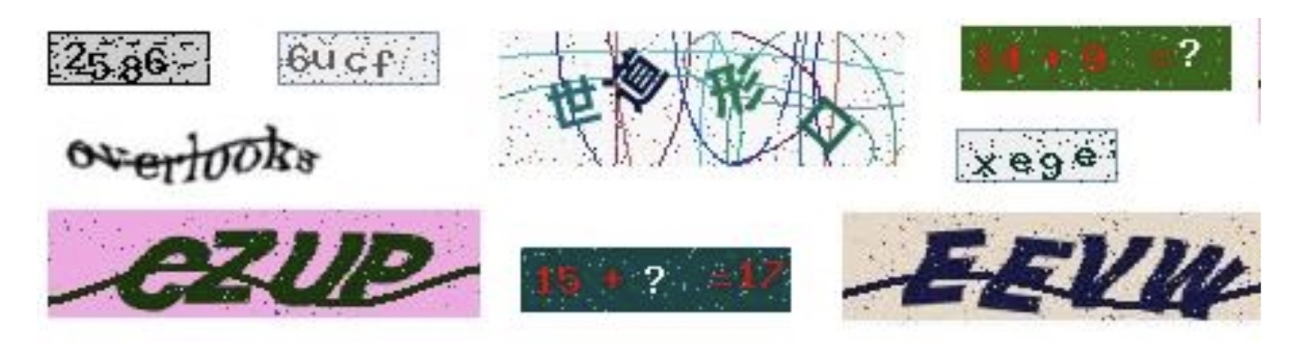3.png

## 验证码识别

### 1. 简单验证码识别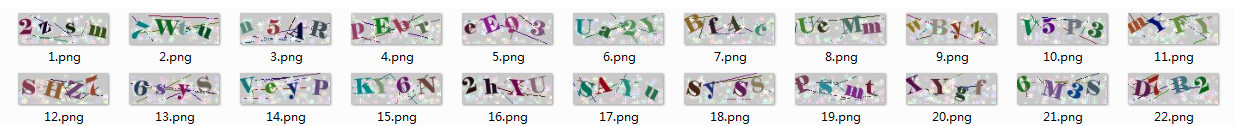4.png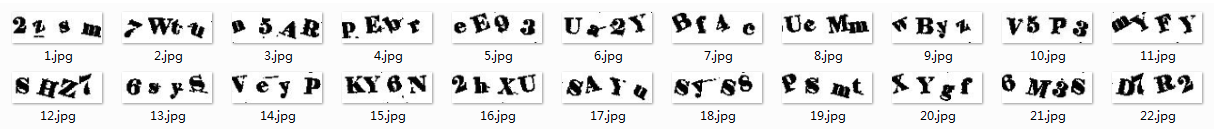5.png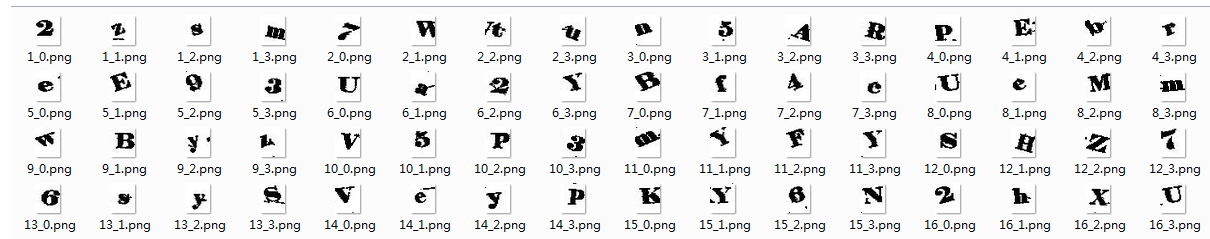6.png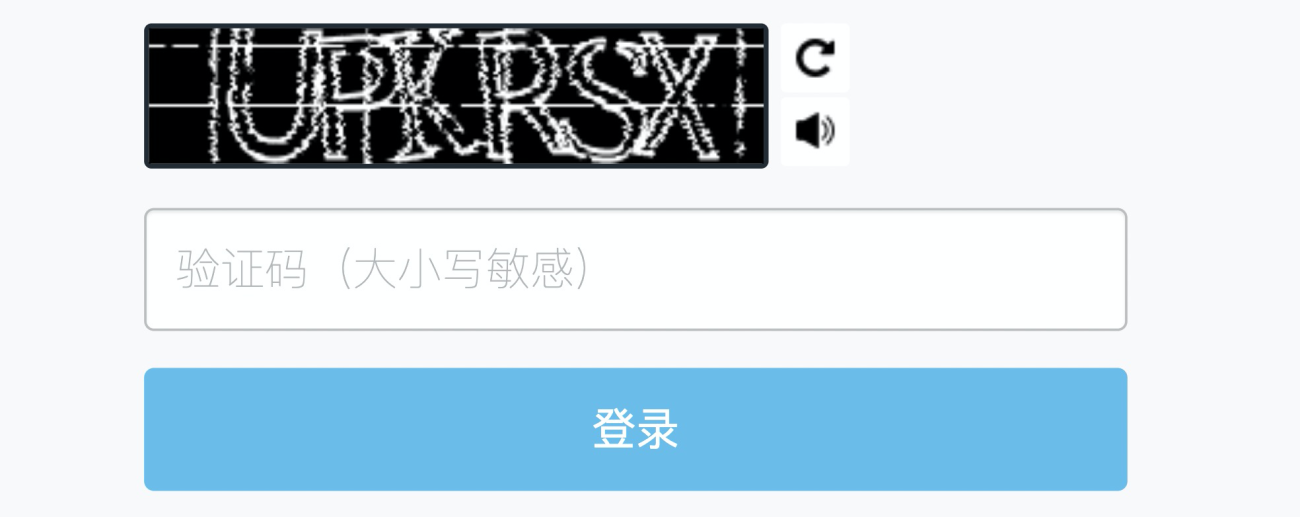7.png

### 2. 基于 CNN 的验证码识别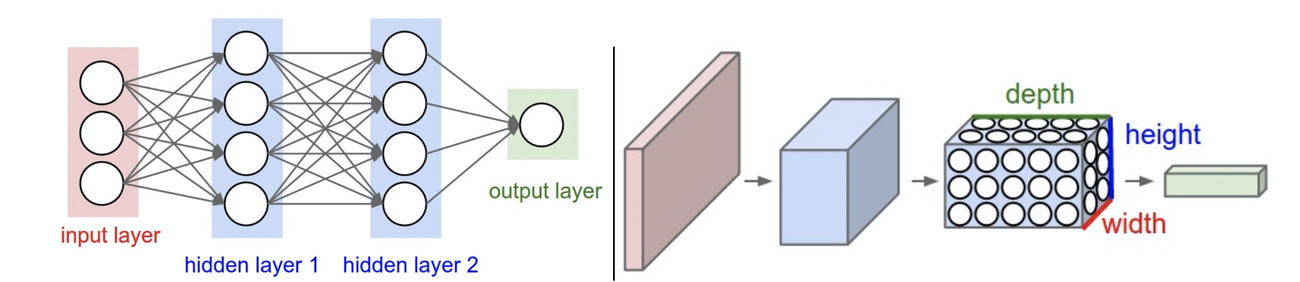8.png

#### 1）验证码生成

# coding:utf-8import randomimport numpy as npfrom PIL import Imagefrom captcha.image import ImageCaptchaCAPTCHA_LIST = [eve for eve in "0123456789abcdefghijklmnopqrsruvwxyzABCDEFGHIJKLMOPQRSTUVWXYZ"]CAPTCHA_LEN = 4  # 验证码长度CAPTCHA_HEIGHT = 60  # 验证码高度CAPTCHA_WIDTH = 160  # 验证码宽度randomCaptchaText = lambda char=CAPTCHA_LIST, size=CAPTCHA_LEN: "".join([random.choice(char) for _ in range(size)])def genCaptchaTextImage(width=CAPTCHA_WIDTH, height=CAPTCHA_HEIGHT, save=None):    image = ImageCaptcha(width=width, height=height)    captchaText = randomCaptchaText()    if save:        image.write(captchaText, './img/%s.jpg' % captchaText)    return captchaText, np.array(Image.open(image.generate(captchaText)))print(genCaptchaTextImage(save=True))image.gif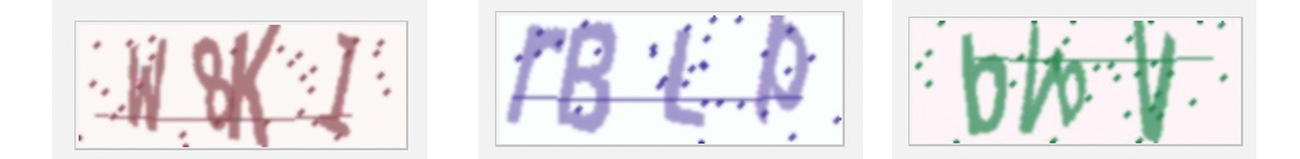9.png

2）模型训练

util.py 文件，主要是一些提取出来的公有方法：

# -*- coding:utf-8 -*-import numpy as npfrom captcha_gen import genCaptchaTextImagefrom captcha_gen import CAPTCHA_LIST, CAPTCHA_LEN, CAPTCHA_HEIGHT, CAPTCHA_WIDTH# 图片转为黑白，3维转1维convert2Gray = lambda img: np.mean(img, -1) if len(img.shape) > 2 else img# 验证码向量转为文本vec2Text = lambda vec, captcha_list=CAPTCHA_LIST: ''.join([captcha_list[int(v)] for v in vec])def text2Vec(text, captchaLen=CAPTCHA_LEN, captchaList=CAPTCHA_LIST):    """    验证码文本转为向量    """    vector = np.zeros(captchaLen * len(captchaList))    for i in range(len(text)):        vector[captchaList.index(text[i]) + i * len(captchaList)] = 1    return vectordef getNextBatch(batchCount=60, width=CAPTCHA_WIDTH, height=CAPTCHA_HEIGHT):    """    获取训练图片组    """    batchX = np.zeros([batchCount, width * height])    batchY = np.zeros([batchCount, CAPTCHA_LEN * len(CAPTCHA_LIST)])    for i in range(batchCount):        text, image = genCaptchaTextImage()        image = convert2Gray(image)        # 将图片数组一维化 同时将文本也对应在两个二维组的同一行        batchX[i, :] = image.flatten() / 255        batchY[i, :] = text2Vec(text)    return batchX, batchY# print(getNextBatch(batch_count=1))

model_train.py 文件，主要是进行模型训练。在该文件中，定义了模型的基本信息，例如该模型是三层卷积神经网络，原始图像大小是 60160，在第一次卷积后变为 60160， 第一池化后变为 3080；第二次卷积后变为 3080 ，第二次池化后变为 1540；第三次卷积后变为  1540 ，第三次池化后变为 720。经过三次卷积和池化后，原始图片数据变为 720 的平面数据，同时项目在进行训练的时候，每隔 100 次进行一次数据测试，计算一次准确度：

# -*- coding:utf-8 -*-import tensorflow.compat.v1 as tffrom datetime import datetimefrom util import getNextBatchfrom captcha_gen import CAPTCHA_HEIGHT, CAPTCHA_WIDTH, CAPTCHA_LEN, CAPTCHA_LISTtf.compat.v1.disable_eager_execution()variable = lambda shape, alpha=0.01: tf.Variable(alpha * tf.random_normal(shape))conv2d = lambda x, w: tf.nn.conv2d(x, w, strides=[1, 1, 1, 1], padding='SAME')maxPool2x2 = lambda x: tf.nn.max_pool(x, ksize=[1, 2, 2, 1], strides=[1, 2, 2, 1], padding='SAME')optimizeGraph = lambda y, y_conv: tf.train.AdamOptimizer(1e-3).minimize(    tf.reduce_mean(tf.nn.sigmoid_cross_entropy_with_logits(labels=y, logits=y_conv)))hDrop = lambda image, weight, bias, keepProb: tf.nn.dropout(    maxPool2x2(tf.nn.relu(conv2d(image, variable(weight, 0.01)) + variable(bias, 0.1))), keepProb)def cnnGraph(x, keepProb, size, captchaList=CAPTCHA_LIST, captchaLen=CAPTCHA_LEN):    """    三层卷积神经网络    """    imageHeight, imageWidth = size    xImage = tf.reshape(x, shape=[-1, imageHeight, imageWidth, 1])    hDrop1 = hDrop(xImage, [3, 3, 1, 32], , keepProb)    hDrop2 = hDrop(hDrop1, [3, 3, 32, 64], , keepProb)    hDrop3 = hDrop(hDrop2, [3, 3, 64, 64], , keepProb)    # 全连接层    imageHeight = int(hDrop3.shape)    imageWidth = int(hDrop3.shape)    wFc = variable([imageHeight * imageWidth * 64, 1024], 0.01)  # 上一层有64个神经元 全连接层有1024个神经元    bFc = variable(, 0.1)    hDrop3Re = tf.reshape(hDrop3, [-1, imageHeight * imageWidth * 64])    hFc = tf.nn.relu(tf.matmul(hDrop3Re, wFc) + bFc)    hDropFc = tf.nn.dropout(hFc, keepProb)    # 输出层    wOut = variable([1024, len(captchaList) * captchaLen], 0.01)    bOut = variable([len(captchaList) * captchaLen], 0.1)    yConv = tf.matmul(hDropFc, wOut) + bOut    return yConvdef accuracyGraph(y, yConv, width=len(CAPTCHA_LIST), height=CAPTCHA_LEN):    """    偏差计算图，正确值和预测值，计算准确度    """    maxPredictIdx = tf.argmax(tf.reshape(yConv, [-1, height, width]), 2)    maxLabelIdx = tf.argmax(tf.reshape(y, [-1, height, width]), 2)    correct = tf.equal(maxPredictIdx, maxLabelIdx)  # 判断是否相等    return tf.reduce_mean(tf.cast(correct, tf.float32))def train(height=CAPTCHA_HEIGHT, width=CAPTCHA_WIDTH, ySize=len(CAPTCHA_LIST) * CAPTCHA_LEN):    """    cnn训练    """    accRate = 0.95    x = tf.placeholder(tf.float32, [None, height * width])    y = tf.placeholder(tf.float32, [None, ySize])    keepProb = tf.placeholder(tf.float32)    yConv = cnnGraph(x, keepProb, (height, width))    optimizer = optimizeGraph(y, yConv)    accuracy = accuracyGraph(y, yConv)    saver = tf.train.Saver()    with tf.Session() as sess:        sess.run(tf.global_variables_initializer())  # 初始化        step = 0  # 步数        while True:            batchX, batchY = getNextBatch(64)            sess.run(optimizer, feed_dict={x: batchX, y: batchY, keepProb: 0.75})            # 每训练一百次测试一次            if step % 100 == 0:                batchXTest, batchYTest = getNextBatch(100)                acc = sess.run(accuracy, feed_dict={x: batchXTest, y: batchYTest, keepProb: 1.0})                print(datetime.now().strftime('%c'), ' step:', step, ' accuracy:', acc)                # 准确率满足要求，保存模型                if acc > accRate:                    modelPath = "./model/captcha.model"                    saver.save(sess, modelPath, global_step=step)                    accRate += 0.01                    if accRate > 0.90:                        break            step = step + 1train()

if accRate > 0.90:    break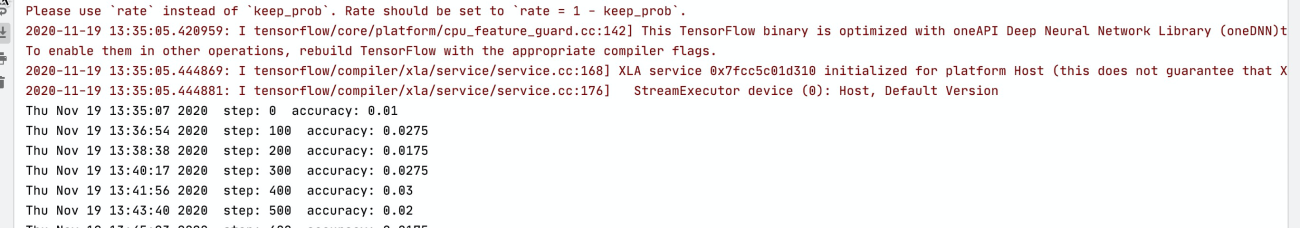10.png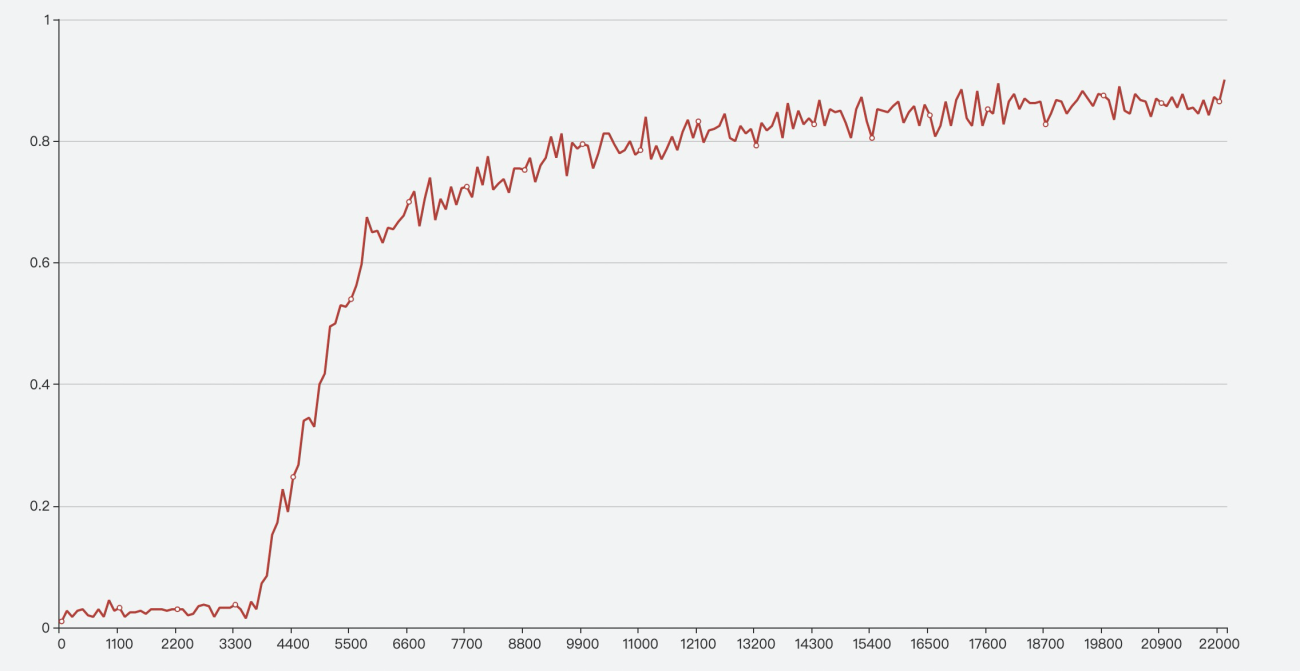11.png

### 3. 基于 Serverless 架构的验证码识别

# -*- coding:utf-8 -*-# 核心后端服务import base64import jsonimport uuidimport tensorflow as tfimport randomimport numpy as npfrom PIL import Imagefrom captcha.image import ImageCaptcha# Responseclass Response:    def __init__(self, start_response, response, errorCode=None):        self.start = start_response        responseBody = {            'Error': {"Code": errorCode, "Message": response},        } if errorCode else {            'Response': response        }        # 默认增加uuid，便于后期定位        responseBody['ResponseId'] = str(uuid.uuid1())        print("Response: ", json.dumps(responseBody))        self.response = json.dumps(responseBody)    def __iter__(self):        status = '200'        response_headers = [('Content-type', 'application/json; charset=UTF-8')]        self.start(status, response_headers)        yield self.response.encode("utf-8")CAPTCHA_LIST = [eve for eve in "0123456789abcdefghijklmnopqrsruvwxyzABCDEFGHIJKLMOPQRSTUVWXYZ"]CAPTCHA_LEN = 4  # 验证码长度CAPTCHA_HEIGHT = 60  # 验证码高度CAPTCHA_WIDTH = 160  # 验证码宽度# 随机字符串randomStr = lambda num=5: "".join(random.sample('abcdefghijklmnopqrstuvwxyz', num))randomCaptchaText = lambda char=CAPTCHA_LIST, size=CAPTCHA_LEN: "".join([random.choice(char) for _ in range(size)])# 图片转为黑白，3维转1维convert2Gray = lambda img: np.mean(img, -1) if len(img.shape) > 2 else img# 验证码向量转为文本vec2Text = lambda vec, captcha_list=CAPTCHA_LIST: ''.join([captcha_list[int(v)] for v in vec])variable = lambda shape, alpha=0.01: tf.Variable(alpha * tf.random_normal(shape))conv2d = lambda x, w: tf.nn.conv2d(x, w, strides=[1, 1, 1, 1], padding='SAME')maxPool2x2 = lambda x: tf.nn.max_pool(x, ksize=[1, 2, 2, 1], strides=[1, 2, 2, 1], padding='SAME')optimizeGraph = lambda y, y_conv: tf.train.AdamOptimizer(1e-3).minimize(    tf.reduce_mean(tf.nn.sigmoid_cross_entropy_with_logits(labels=y, logits=y_conv)))hDrop = lambda image, weight, bias, keepProb: tf.nn.dropout(    maxPool2x2(tf.nn.relu(conv2d(image, variable(weight, 0.01)) + variable(bias, 0.1))), keepProb)def genCaptchaTextImage(width=CAPTCHA_WIDTH, height=CAPTCHA_HEIGHT, save=None):    image = ImageCaptcha(width=width, height=height)    captchaText = randomCaptchaText()    if save:        image.write(captchaText, save)    return captchaText, np.array(Image.open(image.generate(captchaText)))def text2Vec(text, captcha_len=CAPTCHA_LEN, captcha_list=CAPTCHA_LIST):    """    验证码文本转为向量    """    vector = np.zeros(captcha_len * len(captcha_list))    for i in range(len(text)):        vector[captcha_list.index(text[i]) + i * len(captcha_list)] = 1    return vectordef getNextBatch(batch_count=60, width=CAPTCHA_WIDTH, height=CAPTCHA_HEIGHT):    """    获取训练图片组    """    batch_x = np.zeros([batch_count, width * height])    batch_y = np.zeros([batch_count, CAPTCHA_LEN * len(CAPTCHA_LIST)])    for i in range(batch_count):        text, image = genCaptchaTextImage()        image = convert2Gray(image)        # 将图片数组一维化 同时将文本也对应在两个二维组的同一行        batch_x[i, :] = image.flatten() / 255        batch_y[i, :] = text2Vec(text)    return batch_x, batch_ydef cnnGraph(x, keepProb, size, captchaList=CAPTCHA_LIST, captchaLen=CAPTCHA_LEN):    """    三层卷积神经网络    """    imageHeight, imageWidth = size    xImage = tf.reshape(x, shape=[-1, imageHeight, imageWidth, 1])    hDrop1 = hDrop(xImage, [3, 3, 1, 32], , keepProb)    hDrop2 = hDrop(hDrop1, [3, 3, 32, 64], , keepProb)    hDrop3 = hDrop(hDrop2, [3, 3, 64, 64], , keepProb)    # 全连接层    imageHeight = int(hDrop3.shape)    imageWidth = int(hDrop3.shape)    wFc = variable([imageHeight * imageWidth * 64, 1024], 0.01)  # 上一层有64个神经元 全连接层有1024个神经元    bFc = variable(, 0.1)    hDrop3Re = tf.reshape(hDrop3, [-1, imageHeight * imageWidth * 64])    hFc = tf.nn.relu(tf.matmul(hDrop3Re, wFc) + bFc)    hDropFc = tf.nn.dropout(hFc, keepProb)    # 输出层    wOut = variable([1024, len(captchaList) * captchaLen], 0.01)    bOut = variable([len(captchaList) * captchaLen], 0.1)    yConv = tf.matmul(hDropFc, wOut) + bOut    return yConvdef captcha2Text(image_list):    """    验证码图片转化为文本    """    with tf.Session() as sess:        saver.restore(sess, tf.train.latest_checkpoint('model/'))        predict = tf.argmax(tf.reshape(yConv, [-1, CAPTCHA_LEN, len(CAPTCHA_LIST)]), 2)        vector_list = sess.run(predict, feed_dict={x: image_list, keepProb: 1})        vector_list = vector_list.tolist()        text_list = [vec2Text(vector) for vector in vector_list]        return text_listx = tf.placeholder(tf.float32, [None, CAPTCHA_HEIGHT * CAPTCHA_WIDTH])keepProb = tf.placeholder(tf.float32)yConv = cnnGraph(x, keepProb, (CAPTCHA_HEIGHT, CAPTCHA_WIDTH))saver = tf.train.Saver()def handler(environ, start_response):    try:        request_body_size = int(environ.get('CONTENT_LENGTH', 0))    except (ValueError):        request_body_size = 0    requestBody = json.loads(environ['wsgi.input'].read(request_body_size).decode("utf-8"))    imageName = randomStr(10)    imagePath = "/tmp/" + imageName    print("requestBody: ", requestBody)    reqType = requestBody.get("type", None)    if reqType == "get_captcha":        genCaptchaTextImage(save=imagePath)        with open(imagePath, 'rb') as f:            data = base64.b64encode(f.read()).decode()        return Response(start_response, {'image': data})    if reqType == "get_text":        # 图片获取        print("Get pucture")        imageData = base64.b64decode(requestBody["image"])        with open(imagePath, 'wb') as f:            f.write(imageData)        # 开始预测        img = Image.open(imageName)        img = img.resize((160, 60), Image.ANTIALIAS)        img = img.convert("RGB")        img = np.asarray(img)        image = convert2Gray(img)        image = image.flatten() / 255        return Response(start_response, {'result': captcha2Text([image])})

• 获取验证码：用户测试使用，生成验证码

• 获取验证码识别结果：用户识别使用，识别验证码

tensorflow==1.13.1numpy==1.19.4scipy==1.5.4pillow==8.0.1captcha==0.3

# -*- coding:utf-8 -*-import osimport jsonfrom bottle import route, run, static_file, requestimport urllib.requesturl = "http://" + os.environ.get("url")@route('/')def index():    return static_file("index.html", root='html/')@route('/get_captcha')def getCaptcha():    data = json.dumps({"type": "get_captcha"}).encode("utf-8")    reqAttr = urllib.request.Request(data=data, url=url)    return urllib.request.urlopen(reqAttr).read().decode("utf-8")@route('/get_captcha_result', method='POST')def getCaptcha():    data = json.dumps({"type": "get_text", "image": json.loads(request.body.read().decode("utf-8"))["image"]}).encode(        "utf-8")    reqAttr = urllib.request.Request(data=data, url=url)    return urllib.request.urlopen(reqAttr).read().decode("utf-8")run(host='0.0.0.0', debug=False, port=9000)

bottle==0.12.19

<!DOCTYPE html><html lang="en"><head>    <meta charset="UTF-8">    <title>验证码识别测试系统</title>    <link href="https://www.bootcss.com/p/layoutit/css/bootstrap-combined.min.css" rel="stylesheet">    <script>        var image = undefined        function getCaptcha() {            const xmlhttp = window.XMLHttpRequest ? new XMLHttpRequest() : new ActiveXObject("Microsoft.XMLHTTP");            xmlhttp.open("GET", '/get_captcha', false);            xmlhttp.onreadystatechange = function () {                if (xmlhttp.readyState == 4 && xmlhttp.status == 200) {                    image = JSON.parse(xmlhttp.responseText).Response.image                    document.getElementById("captcha").src = "data:image/png;base64," + image                    document.getElementById("getResult").style.visibility = 'visible'                }            }            xmlhttp.setRequestHeader("Content-type", "application/json");            xmlhttp.send();        }        function getCaptchaResult() {            const xmlhttp = window.XMLHttpRequest ? new XMLHttpRequest() : new ActiveXObject("Microsoft.XMLHTTP");            xmlhttp.open("POST", '/get_captcha_result', false);            xmlhttp.onreadystatechange = function () {                if (xmlhttp.readyState == 4 && xmlhttp.status == 200) {                    document.getElementById("result").innerText = "识别结果：" + JSON.parse(xmlhttp.responseText).Response.result                }            }            xmlhttp.setRequestHeader("Content-type", "application/json");            xmlhttp.send(JSON.stringify({"image": image}));        }    </script></head><body><div class="container-fluid" style="margin-top: 10px">    <div class="row-fluid">        <div class="span12">            <center>                <h3>                    验证码识别测试系统                </h3>            </center>        </div>    </div>    <div class="row-fluid">        <div class="span2">        </div>        <div class="span8">            <center>                <img src="" id="captcha"/>                <br><br>                <p id="result"></p>            </center>            <fieldset>                <legend>操作：</legend>                <button class="btn" onclick="getCaptcha()">获取验证码</button>                <button class="btn" onclick="getCaptchaResult()" id="getResult" style="visibility: hidden">识别验证码                </button>            </fieldset>        </div>        <div class="span2">        </div>    </div></div></body></html>

Global:  Service:      Name: ServerlessBook      Description: Serverless图书案例      Log: Auto      Nas: AutoServerlessBookCaptchaDemo:  Component: fc  Provider: alibaba  Access: release  Extends:    deploy:      - Hook: s install docker        Path: ./        Pre: true  Properties:    Region: cn-beijing    Service: ${Global.Service} Function: Name: serverless_captcha Description: 验证码识别 CodeUri: Src: ./src/backend Excludes: - src/backend/.fun - src/backend/model Handler: index.handler Environment: - Key: PYTHONUSERBASE Value: /mnt/auto/.fun/python MemorySize: 3072 Runtime: python3 Timeout: 60 Triggers: - Name: ImageAI Type: HTTP Parameters: AuthType: ANONYMOUS Methods: - GET - POST - PUT Domains: - Domain: AutoServerlessBookCaptchaWebsiteDemo: Component: bottle Provider: alibaba Access: release Extends: deploy: - Hook: pip3 install -r requirements.txt -t ./ Path: ./src/website Pre: true Properties: Region: cn-beijing CodeUri: ./src/website App: index.py Environment: - Key: url Value:${ServerlessBookCaptchaDemo.Output.Triggers.Domains}    Detail:      Service: \${Global.Service}      Function:        Name: serverless_captcha_website

| - src # 项目目录 |   | - backend # 项目后端，核心接口 |       | - index.py # 后端核心代码 |       | - requirements.txt # 后端核心代码依赖 |   | - website # 项目前端，便于测试使用 |       | - html # 项目前端页面 |           | - index.html # 项目前端页面 |       | - index.py # 项目前端的后台服务（bottle框架） |       | - requirements.txt # 项目前端的后台服务依赖

s deploy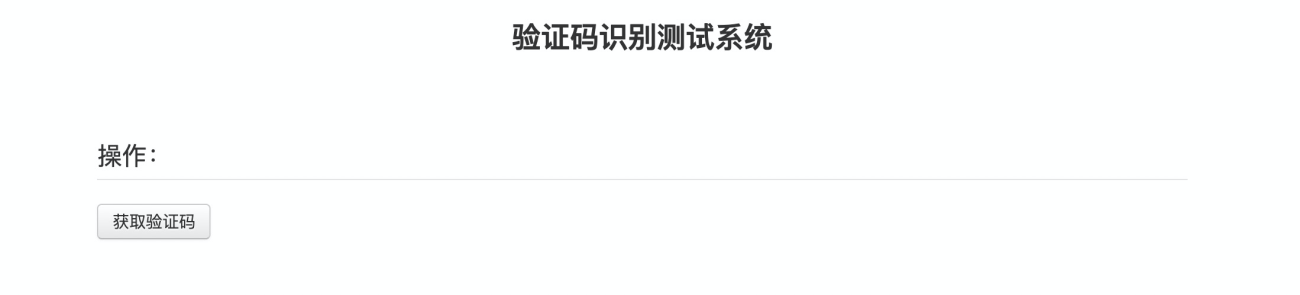12.png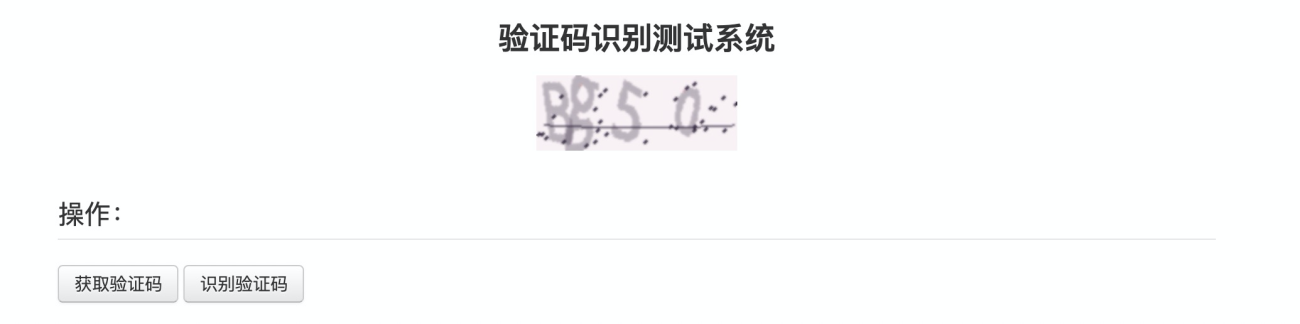13.png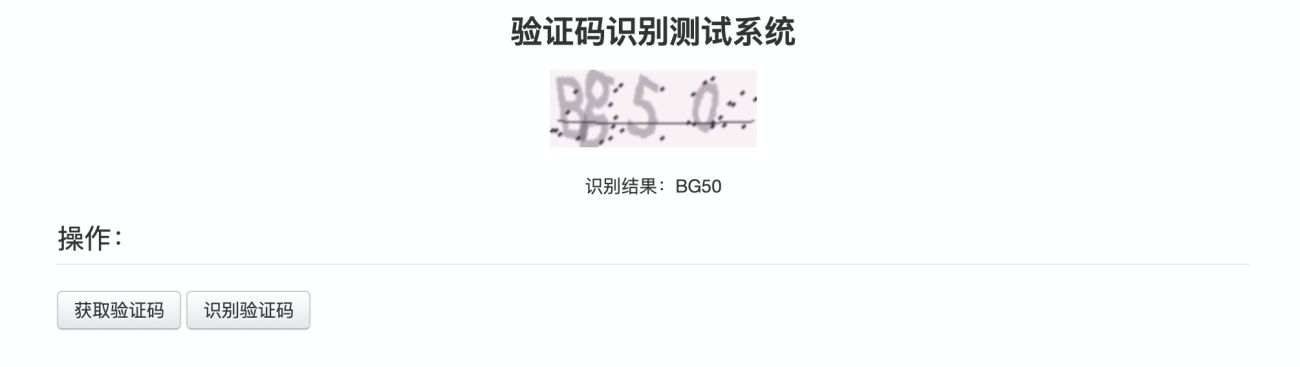14.png

## 总结

Serverless 发展迅速，通过 Serverless 做一个验证码识别工具，我觉得这是一个非常酷的事情。在未来的数据采集等工作中，有一个优美的验证码识别工具是非常必要的。当然验证码种类很多，针对不同类型的验证码识别，也是一项非常有挑战性的工作。## 评论